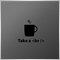# Average order price65

I Try to calculate average price of open orders . I am unebel to calculate it. I don't know  reason. I am very thankful if eny one can helpme

```   for(int i=0; i<OrdersTotal(); i++)
{
break;
if(OrderSymbol()==Symbol() && OrderMagicNumber()==MAGICMA)
if(OrderType()==OP_SELL)
{
Lot_Price+=OrderLots()*OrderOpenPrice();
Total_Lots+=OrderLots();

Total_Orders=Total_Orders +1;
Sell_Profit=Sell_Profit+OrderProfit()+OrderCommission()+OrderSwap();
}

}

Avg_Sell=NormalizeDouble((Lot_Price/Total_Lots),Digits);```

RGD51

I Try to calculate average price of open orders . I am unebel to calculate it. I don't know  reason. I am very thankful if eny one can helpme

RGD

`           Lot_Price+=Lot_Price+(OrderLots()*OrderOpenPrice());`
maybe...21958

1. roshjardine: maybe...
`        Lot_Price+=Lot_Price+(OrderLots()*OrderOpenPrice());`

Absolutely not.

1. manntrade: I Try to calculate average price of open orders . I am unebel to calculate it. I don't know  reason. I am very thankful if eny one can helpme

You correctly calculated lots weighted average open price; that is your break even price. You were. There is no reason. There is no one able.

2. Why did you post your MT4 question in the Root / MT5 General section instead of the MQL4 section, (bottom of the Root page?)
General rules and best pratices of the Forum. - General - MQL5 programming forum
Next time post in the correct place. The moderators will likely move this thread there soon.51

William Roeder:
1. Absolutely not.

1. You correctly calculated lots weighted average open price; that is your break even price. You were. There is no reason. There is no one able.

2. Why did you post your MT4 question in the Root / MT5 General section instead of the MQL4 section, (bottom of the Root page?)
General rules and best pratices of the Forum. - General - MQL5 programming forum
Next time post in the correct place. The moderators will likely move this thread there soon.

you are right William..thanks for pointing the mistake...65

roshjardine:
maybe...

Thanks for the respons. Lot_Price is correct.65

William Roeder:
1. Absolutely not.

1. You correctly calculated lots weighted average open price; that is your break even price. You were. There is no reason. There is no one able.

2. Why did you post your MT4 question in the Root / MT5 General section instead of the MQL4 section, (bottom of the Root page?)
General rules and best pratices of the Forum. - General - MQL5 programming forum
Next time post in the correct place. The moderators will likely move this thread there soon.

Sorry for the mistake

`Avg_Sell=NormalizeDouble((Lot_Price/Total_Lots),Digits);`

if this line is there. EA is not runnig.65

Thank you every one Problem solved

Problem is dividing zero value. now i have avoided dividing the zero value

RGD